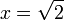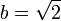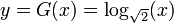# Equation (mathematics)

In mathematics, an equation is a statement that two quantities are equal. It is usually regarded as a kind of mathematical problem in which you have to find a value which makes the equation true. A simple example is the question: What do you have to fill in on the dots in … + 2 = 3? The answer is 1, because 1 + 2 = 3. The unknown quantity in such problems is frequently denoted by a letter, often x, so that the equation becomes x + 2 = 3. The solution of this equation is x = 1. In general, an equation may have no solution, one solution or many solutions.

By contrast, an identity is an equality which is stated to be universally true for all permissible values of the variables, rather than representing a condition on those values. For example, x + y = y + x is an identity for real numbers, since it is true for all real values of x and y.

Scientific laws are often formulated as equations, especially in physics and other natural sciences. Examples are Newton's laws, the equation of a harmonic oscillator and the Schrödinger equation. The term equation is used also in chemistry, indicating conservation of atomic or isotopic content (and rarely other forms of energy, e.g. light) during chemical reactions.

## Domain of the unknown

Technically, an equation has to indicate what values the unknown variable can take. This is called the domain of the variable. For instance, the equation x + 1 = 0 has no solutions if x is supposed to be a natural number, but it does have a solution (namely, x = −1) if x is supposed to be an integer number.

Similarly, the equation x2 = 2 has no solutions if the domain is formed by the rational numbers, it has two solutions (namely,$x=\sqrt{2}$ and$x=-\sqrt{2}$) among the real numbers, and it has only one solution (namely,$x=\sqrt{2}$) among the positive real numbers.

Often, the domain is not specified explicitly, but it is assumed that the reader knows what it is supposed to be.

## Inverse function

The equation may have the form

(1) F(x) = 0

or

(2) F(x) = G(x)

where F and G are known functions and x is the unknown variable.

Function F in equation (1) ; or functions F and G in equation (2) may also depend on some parameter(s). In this case, the solution(s) x also may depend on parameter(s). Indicating the function, the parameter, say, b, can be specified as a second argument, writing F(x,b), G(x,b) or as subscript, writing Fb(x), Gb(x).

In relatively simple cases, function F depends only on the unknown variable, and G depends on the parameter; for example,

(3) F(x) = b .

In this case, the solution x is considered as an inverse function of b, which can be written as

(4) x = F − 1(b).

Depending on the function F, range of values of b and the domain of x, there may exist no inverse function, one inverse function or several inverse functions.

## Graphical solution of equationsFig.1. Example of graphic solution of equation x = logb(x) for$b=\sqrt{2}$ (two solutions, x = 2 and x = 4), b = exp(1 / e) (one solution x = e), and b = 2 (no real solutions).

Solving equations, it may worth to begin with graphic solution of the equation, which allows the quick and dirty estimates. One plots both functions, F and G at the same graphic, and watch the point (s) of the intersection of the curves. In figure 1, functions y = F(x) = x is plotted with black line, and function$y=G(x)=\log_{\sqrt{2}}(x)$ is plotted with red curve. The intersections with black curve indicate values of x which are solutions.

At the same figure, the cases G(x) = logexp(1 / e)(x) (only one solution, x = e) and G(x) = log2(x) (no solutions among real numbers) are shown with green and blue curves.

## System of equations

In the equations (1) or (2), x may denote several numbers at once, x = {x0,x1,..xn − 1}; and functions F and G may return values from multidimensional space {F0,F1,F2,..Fm − 1}. In this case, one says that there is system of equations. For example, there is well developed theory of systems of linear equations, while unknown variables x are real or complex numbers.

## Differential equations and integral equations

In particular, the variable may denote a function of one or several variables, so that the set of possible values is some functional space, usually a Banach or even Hilbert space; the function F is then an operator on this space which may be expressed in terms of derivatives or integrals of the function elements. In these cases, the equation is called a differential equation or an integral equation.

## Operator equations

Equations can be used for objects of any origin, as soon, as the operation of equality is defined. In particular, in Quantum mechanics, the Heisenberg equation deals with non-commuting objects (operators).Some content on this page may previously have appeared on Citizendium.